# IBM HR Analytics Employee Attrition & Performance using KNN

Attrition is a problem that impacts all businesses, irrespective of geography, industry and size of the company. It is a major problem to an organization, and predicting turnover is at the forefront of the needs of Human Resources (HR) in many organizations. Organizations face huge costs resulting from employee turnover. With advances in machine learning and data science, it’s possible to predict the employee attrition and we will predict using KNN (k-nearest neighbours) algorithm.

Dataset:
The dataset that is published by the Human Resource department of IBM is made available at Kaggle.
dataset

Code: Implementation of KNN algorithm for classification.

 `# performing linear algebra ` `import` `numpy as np  ` ` `  `# data processing ` `import` `pandas as pd ` ` `  `# visualisation ` `import` `matplotlib.pyplot as plt ` `import` `seaborn as sns ``%` `matplotlib inline `

Code: Importing the dataset

 `dataset ``=` `pd.read_csv(``"WA_Fn-UseC_-HR-Employee-Attrition.csv"``) ` `print` `(dataset.head) `

Output :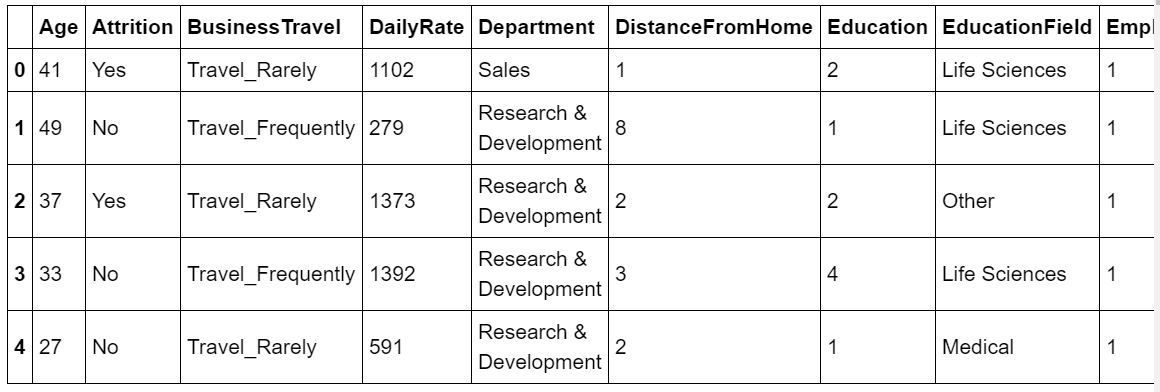`df.info() `

Output :

```
RangeIndex: 1470 entries, 0 to 1469
Data columns (total 35 columns):
Age                         1470 non-null int64
Attrition                   1470 non-null object
DailyRate                   1470 non-null int64
Department                  1470 non-null object
DistanceFromHome            1470 non-null int64
Education                   1470 non-null int64
EducationField              1470 non-null object
EmployeeCount               1470 non-null int64
EmployeeNumber              1470 non-null int64
EnvironmentSatisfaction     1470 non-null int64
Gender                      1470 non-null object
HourlyRate                  1470 non-null int64
JobInvolvement              1470 non-null int64
JobLevel                    1470 non-null int64
JobRole                     1470 non-null object
JobSatisfaction             1470 non-null int64
MaritalStatus               1470 non-null object
MonthlyIncome               1470 non-null int64
MonthlyRate                 1470 non-null int64
NumCompaniesWorked          1470 non-null int64
Over18                      1470 non-null object
OverTime                    1470 non-null object
PercentSalaryHike           1470 non-null int64
PerformanceRating           1470 non-null int64
RelationshipSatisfaction    1470 non-null int64
StandardHours               1470 non-null int64
StockOptionLevel            1470 non-null int64
TotalWorkingYears           1470 non-null int64
TrainingTimesLastYear       1470 non-null int64
WorkLifeBalance             1470 non-null int64
YearsAtCompany              1470 non-null int64
YearsInCurrentRole          1470 non-null int64
YearsWithCurrManager        1470 non-null int64
dtypes: int64(26), object(9)
memory usage: 402.0+ KB
```

Cdoe: Visualizing the data

 `# heatmap to check the missing value ` `plt.figure(figsize ``=``(``10``, ``4``)) ` `sns.heatmap(dataset.isnull(), yticklabels ``=` `False``, cbar ``=` `False``, cmap ``=``'viridis'``) `

Output: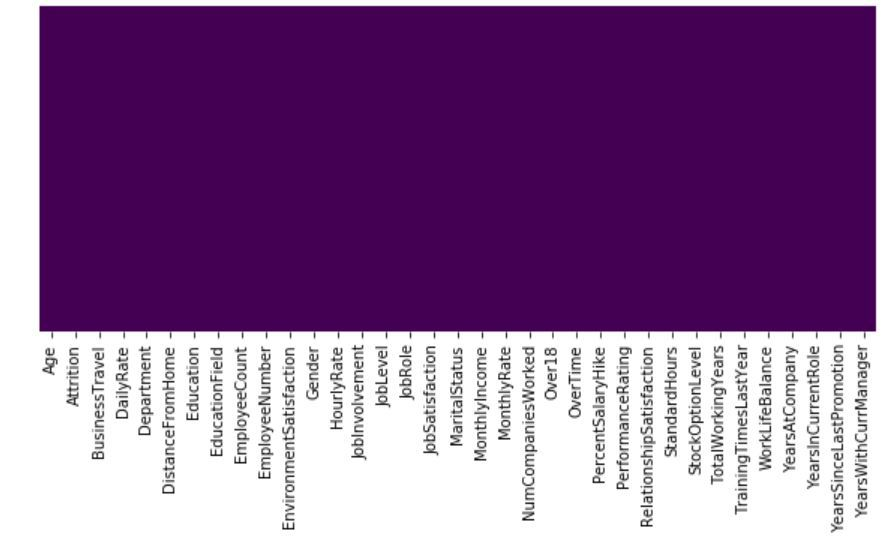So, we can see that there are no missing values in the dataset.
This is a Binary Classification Problem, so the Distribution of instances among the 2 classes, is visualized below:

 `sns.set_style(``'darkgrid'``) ` `sns.countplot(x ``=``'Attrition'``, data ``=` `dataset) `

Output: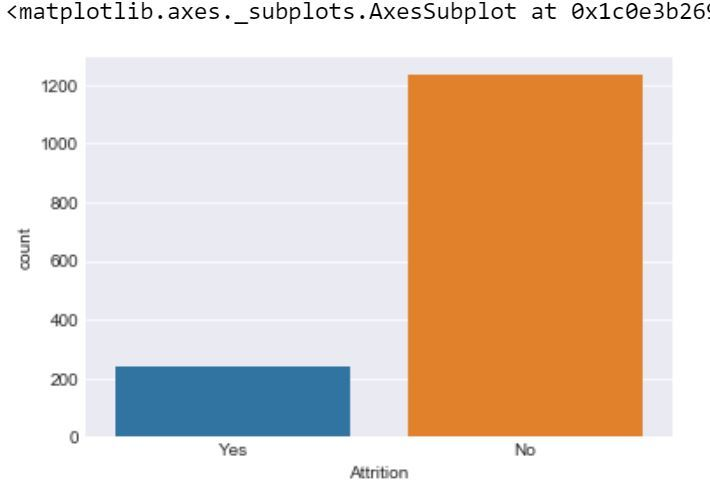Code:

 `sns.lmplot(x ``=` `'Age'``, y ``=` `'DailyRate'``, hue ``=` `'Attrition'``, data ``=` `dataset)  `

Output:

```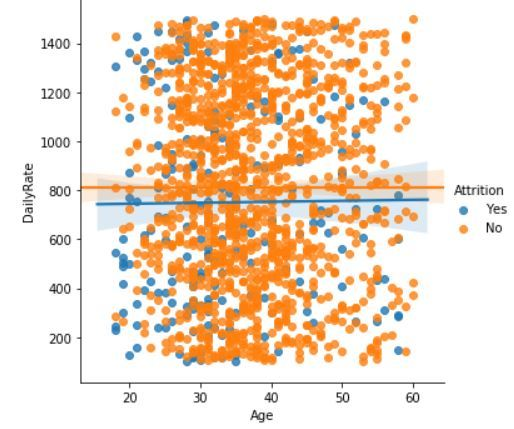```

Code :

 `plt.figure(figsize ``=``(``10``, ``6``)) ` `sns.boxplot(y ``=``'MonthlyIncome'``, x ``=``'Attrition'``, data ``=` `dataset)`

Output:

```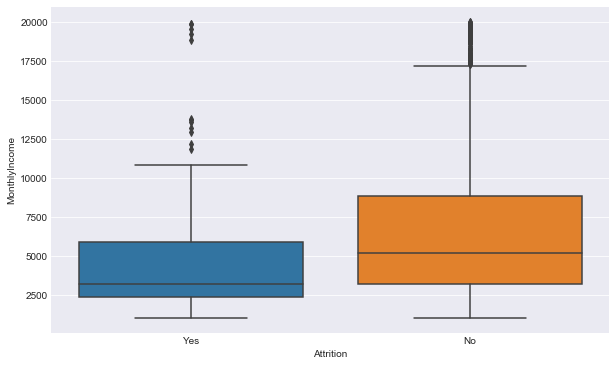```

Preprocessing the data
In the dataset there are 4 irrelevant columns, i.e:EmployeeCount, EmployeeNumber, Over18 and StandardHour. So, we have to remove these for more accuracy.
Code:

 `dataset.drop(``'EmployeeCount'``, axis ``=` `1``, inplace ``=` `True``) ` `dataset.drop(``'StandardHours'``, axis ``=` `1``, inplace ``=` `True``) ` `dataset.drop(``'EmployeeNumber'``, axis ``=` `1``, inplace ``=` `True``) ` `dataset.drop(``'Over18'``, axis ``=` `1``, inplace ``=` `True``) ` `print``(dataset.shape) `

Output:

```(1470, 31)
```

So, we have removed the irrelevant column.

Code: Input and Output data

 `y ``=` `dataset.iloc[:, ``1``] ` `X ``=` `dataset ` `X.drop(``'Attrition'``, axis ``=` `1``, inplace ``=` `True``) `

Code: Label Encoding

 `from` `sklearn.preprocessing ``import` `LabelEncoder ` `lb ``=` `LabelEncoder() ` `y ``=` `lb.fit_transform(y) `

In the dataset there are 7 categorical data, so we have to change them to int data, i.e we hava to create 7 dummy variable for better accuracy.

Code: Dummy variable creation

 `dum_BusinessTravel ``=` `pd.get_dummies(dataset[``'BusinessTravel'``],  ` `                                    ``prefix ``=``'BusinessTravel'``) ` `dum_Department ``=` `pd.get_dummies(dataset[``'Department'``],  ` `                                ``prefix ``=``'Department'``) ` `dum_EducationField ``=` `pd.get_dummies(dataset[``'EducationField'``],  ` `                                    ``prefix ``=``'EducationField'``) ` `dum_Gender ``=` `pd.get_dummies(dataset[``'Gender'``],  ` `                            ``prefix ``=``'Gender'``, drop_first ``=` `True``) ` `dum_JobRole ``=` `pd.get_dummies(dataset[``'JobRole'``],  ` `                             ``prefix ``=``'JobRole'``) ` `dum_MaritalStatus ``=` `pd.get_dummies(dataset[``'MaritalStatus'``],  ` `                                   ``prefix ``=``'MaritalStatus'``) ` `dum_OverTime ``=` `pd.get_dummies(dataset[``'OverTime'``],  ` `                              ``prefix ``=``'OverTime'``, drop_first ``=` `True``) ` `# Adding these dummy variable to input X ` `X ``=` `pd.concat([x, dum_BusinessTravel, dum_Department,  ` `               ``dum_EducationField, dum_Gender, dum_JobRole,  ` `               ``dum_MaritalStatus, dum_OverTime], axis ``=` `1``) ` `# Removing the categorical data ` `X.drop([``'BusinessTravel'``, ``'Department'``, ``'EducationField'``,  ` `        ``'Gender'``, ``'JobRole'``, ``'MaritalStatus'``, ``'OverTime'``],  ` `        ``axis ``=` `1``, inplace ``=` `True``) ` ` `  `print``(X.shape) ` `print``(y.shape) `

Output:

```(1470, 49)
(1470, )

```

Code: Splitting data to training and testing

 `from` `sklearn.model_selection ``import` `train_test_split ` `X_train, X_test, y_train, y_test ``=` `train_test_split( ` `    ``X, y, test_size ``=` `0.25``, random_state ``=` `40``) `

So, the preprocessing is done, now we have to apply KNN to the dataset.
Model Execution code: Using KNeighborsClassifier for finding the best number of neighbour with the help of misclassification error.

 `from` `sklearn.neighbors ``import` `KNeighborsClassifier ` `neighbors ``=` `[]  ` `cv_scores ``=` `[]  ` `   `  `from` `sklearn.model_selection ``import` `cross_val_score  ` `# perform 10 fold cross validation  ` `for` `k ``in` `range``(``1``, ``40``, ``2``):  ` `    ``neighbors.append(k)  ` `    ``knn ``=` `KNeighborsClassifier(n_neighbors ``=` `k)  ` `    ``scores ``=` `cross_val_score(  ` `        ``knn, X_train, y_train, cv ``=` `10``, scoring ``=` `'accuracy'``)  ` `    ``cv_scores.append(scores.mean()) ` `error_rate ``=` `[``1``-``x ``for` `x ``in` `cv_scores]  ` `   `  `# determining the best k  ` `optimal_k ``=` `neighbors[error_rate.index(``min``(error_rate))]  ` `print``(``'The optimal number of neighbors is % d '` `%` `optimal_k)  ` `   `  `# plot misclassification error versus k  ` `plt.figure(figsize ``=` `(``10``, ``6``))  ` `plt.plot(``range``(``1``, ``40``, ``2``), error_rate, color ``=``'blue'``, linestyle ``=``'dashed'``, marker ``=``'o'``, ` `         ``markerfacecolor ``=``'red'``, markersize ``=` `10``) ` `plt.xlabel(``'Number of neighbors'``)  ` `plt.ylabel(``'Misclassification Error'``)  ` `plt.show()  `

Output:

```The optimal number of neighbors is  7
```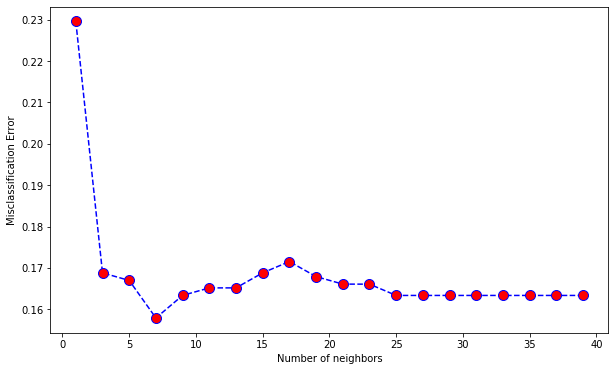Code: Prediction Score

 `from` `sklearn.model_selection ``import` `cross_val_predict, cross_val_score ` `from` `sklearn.metrics ``import` `accuracy_score, classification_report ` `from` `sklearn.metrics ``import` `confusion_matrix ` ` `  `def` `print_score(clf, X_train, y_train, X_test, y_test, train ``=` `True``): ` `    ``if` `train: ` `        ``print``(``"Train Result:"``) ` `        ``print``(``"------------"``) ` `        ``print``(``"Classification Report: \n {}\n"``.``format``(classification_report( ` `                ``y_train, clf.predict(X_train)))) ` `        ``print``(``"Confusion Matrix: \n {}\n"``.``format``(confusion_matrix( ` `                ``y_train, clf.predict(X_train)))) ` ` `  `        ``res ``=` `cross_val_score(clf, X_train, y_train,  ` `                              ``cv ``=` `10``, scoring ``=``'accuracy'``) ` `        ``print``(``"Average Accuracy: \t {0:.4f}"``.``format``(np.mean(res))) ` `        ``print``(``"Accuracy SD: \t\t {0:.4f}"``.``format``(np.std(res))) ` `        ``print``(``"accuracy score: {0:.4f}\n"``.``format``(accuracy_score( ` `                ``y_train, clf.predict(X_train)))) ` `        ``print``(``"----------------------------------------------------------"``) ` `                `  `    ``elif` `train ``=``=` `False``: ` `        ``print``(``"Test Result:"``) ` `        ``print``(``"-----------"``) ` `        ``print``(``"Classification Report: \n {}\n"``.``format``( ` `                ``classification_report(y_test, clf.predict(X_test)))) ` `        ``print``(``"Confusion Matrix: \n {}\n"``.``format``( ` `                ``confusion_matrix(y_test, clf.predict(X_test))))  ` `        ``print``(``"accuracy score: {0:.4f}\n"``.``format``( ` `                ``accuracy_score(y_test, clf.predict(X_test)))) ` `        ``print``(``"-----------------------------------------------------------"``) ` `         `  `knn ``=` `KNeighborsClassifier(n_neighbors ``=` `7``) ` `knn.fit(X_train, y_train) ` `print_score(knn, X_train, y_train, X_test, y_test, train ``=` `True``) ` `print_score(knn, X_train, y_train, X_test, y_test, train ``=` `False``) `

Output:

```Train Result:
------------
Classification Report:
precision    recall  f1-score   support

0       0.86      0.99      0.92       922
1       0.83      0.19      0.32       180

accuracy                           0.86      1102
macro avg       0.85      0.59      0.62      1102
weighted avg       0.86      0.86      0.82      1102

Confusion Matrix:
[[915   7]
[145  35]]

Average Accuracy:      0.8421
Accuracy SD:          0.0148
accuracy score: 0.8621

-----------------------------------------------------------
Test Result:
-----------
Classification Report:
precision    recall  f1-score   support

0       0.84      0.96      0.90       311
1       0.14      0.04      0.06        57

accuracy                           0.82       368
macro avg       0.49      0.50      0.48       368
weighted avg       0.74      0.82      0.77       368

Confusion Matrix:
[[299  12]
[ 55   2]]

accuracy score: 0.8179

```

My Personal Notes arrow_drop_upCheck out this Author's contributed articles.

If you like GeeksforGeeks and would like to contribute, you can also write an article using contribute.geeksforgeeks.org or mail your article to contribute@geeksforgeeks.org. See your article appearing on the GeeksforGeeks main page and help other Geeks.

Please Improve this article if you find anything incorrect by clicking on the "Improve Article" button below.

Article Tags :
Practice Tags :

Be the First to upvote.

Please write to us at contribute@geeksforgeeks.org to report any issue with the above content.